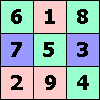ONE TO NINE by Andrew HodgesPage 241: An excellent history and explanation of ASCII is given by Tom Jennings on this page.

Page 246: Ulam's letter was written to me. It is reproduced on this page of my Alan Turing website.

Page 248:

Get each of the numbers in the form 10n.

This is easy for (A), (C), (D), (F), where the powers of 10 are respectively 8 × 108, 100, 10100, 10123.
So C < A < D < F.

For the others, make use of 210 ≈ 103.

(G) is 265536 ≈ 10(0.3 × 65536) ≈ 1020000 and so lies between (C) and (A).

(B) is 272 × 2256 ≈ 272 × 1078 ≈ 1080 and so is less than (C).

(H) is 2 to the power of about 1020000. A rather surprising fact is that because this huge number is 10 to the power of about 0.3 × 1020000, we may as well say this is ≈ 1020000. It is much greater than (F).

What is (E)? The largest number you can make out of 4 4's comes from putting them in a tower of powers, i.e. 4 to the power of (4 to the power of 44). This is 4 to the power of 4256, which is 4 to the power of 2512 ≈ 4 to the power of 10156. Again, this is ≈ 10 to the power of 10156. So (E) is bigger than (F), but not as big as (H).

The final order is B, C, G, A, D, F, E, H.Page 260:

Notice first that 6 and 28 are 110 and 11100 in binary, so fall into this pattern, with 3 (11 in binary) and 7 (111 in binary) as Mersenne primes. Use the number 28 as a model for the general case. See the pattern in the binary. Because 111 is prime, the factors either have 111 in them or not. The factors without a 111 are (1, 10, 100), and these add up to 111. The factors with a 111 are: (111, 1110) and now 111+111+1110=1110+1110=11100 as requred for perfection.

It is known that any even perfect number must be of this form. It is not known whether there exist any odd perfect numbers.

The second question is also easily seen from the binary pattern. The question in Chapter 1 asked for 7 × 9 in binary, giving 111111 representing 63. We can look at this in reverse: because 111111 has six 1's, and six is not a prime, it can be split up as 11-11-11 showing that 11 is a factor, or as 111-111 showing that 111 is a factor. That is, 111111 = 11 × 10101 = 111 × 1001. The same argument works for a string of n 1's whenever n is not prime.

Page 263:

The point is that 65535 = 257 × 255 = 257 × 17 × 15 = 257 × 17 × 5 × 3. So the product 65535 × 65536 × 65537 involves each of the Fermat primes just once, plus 16 2's.Page 266:

The first question just needs a count. Remember that the sequence is a cycle, so the last 0 counts as being followed by 0001... taken from the beginning.

The second question can also be done just by trying them out. But it is more useful to see a pattern in the results. We have already found that modulo 17, the powers of 10 are:
101=10, 102=15, 103=14, 104=4, 105=6, 106=9, 107=5, 108=16,
109=7, 1010=2, 1011=3, 1012=13, 1013=11, 1014=8, 1015=12, 1016=1.

So looking at the powers of 15, modulo 17, is the same as looking at the powers of 102, and clearly these will only give the even powers of 10: 104, 106, 108... 1016.

Similarly for powers of 4, 9, 16, 2, 13, 8. But looking at the powers of 14, modulo 17, is the same as looking at the powers of 103, and these will run through all 16 possibilities. Similarly for all the odd powers of 10.

The last question is just another way of expressing this observation. The numbers 4, 9, 16, 2, 13, 8 are all squares, modulo 17, and so lie on the diagonal of the base-17 multiplication table.

Page 272: Breaking of GSM mobile phone system